PRICE/EARNINGS MULTIPLES

Question 1 - P/E Ratio for a Stable Firm

National City Corporation, a bank holding company, reported earnings per share of \$2.40 in 1993, and paid dividends per share of \$1.06. The earnings had grown 7.5% a year over the prior five years, and were expected to grow 6% a year in the long term (starting in 1994). The stock had a beta of 1.05 and traded for ten times earnings. The treasury bond rate was 7%.

A. Estimate the P/E Ratio for National City Corporation.

B. What long term growth rate is implied in the firm's current P/E ratio?

Question 2 - P/E Ratio for a Market

On March 11, 1994, the New York Stock Exchange Composite was trading at 16.9 times earnings, and the average dividend yield across stocks on the exchange was 2.5%. The treasury bond rate on March 11, 1994, was 6.95%. The economy was expected to grow 2.5% a year, in real terms, in the long term, and the consensus estimate for inflation, in the long term, was 3.5%.

A. Based upon these inputs, estimate the appropriate P/E ratio for the exchange.

B. What growth rate in dividends/earnings would justify the P/E ratio on March 11, 1994?

C. Would it matter whether this higher growth comes from higher inflation or higher real growth? Why?

Question 3 - P/E Ratio for a High Growth Firm

International Flavors and Fragrances, a leading creator and manufacturer of flavors and fragrances, paid out dividends of \$0.91 per share on earnings per share of \$1.64 in 1992. The firm is expected to have a return on equity of 20% between 1993 and 1997, after which the firm is expected to have stable growth of 6% a year (the return on equity is expected to drop to 15% in the stable growth phase.) The dividend payout ratio is expected to remain at the current level from 1993 to 1997. The stock has a beta of 1.10, which is not expected to change. The treasury bond rate is 7%.

A. Estimate the P/E ratio for International Flavors, based upon fundamentals.

B. Estimate how much of this P/E ratio can be ascribed to the extraordinary growth in earnings that the firm expects to have between 1993 and 1997.

Question 4 - P/E Ratio as a Function of Growth

Cracker Barrel, which operates restaurants and gift shops, reported dramatic growth in earnings and revenues between 1983 and 1992. During this period, earnings grew from \$0.08 per share in 1983 to \$0.78 per share in 1993. The dividends paid in 1993 amounted to only \$0.02 per share. The earnings growth rate was expected to ease to 15% a year from 1994 to 1998, and to 6% a year after that. The payout ratio is expected to increase to 10% from 1994 to 1998, and to 50% after that. The beta of the stock is currently 1.55, but it is expected to decline to 1.25 for the 1994-98 time period and to 1.10 after that. The treasury bond rate is 7%.

A. Estimate the P/E ratio for Cracker Barrel.

B. Estimate how much higher the P/E ratio would have been, if it had been able to maintain the growth rate in earnings that it had posted between 1983 and 1993. (Assume that the dividend payout ratios are unaffected.)

C. Now assume that disappointing earnings reports in the near future lower the expected growth rate between 1994 and 1998 to 10%. Estimate the P/E ratio. (Again, assume that the dividend payout ratio is unaffected.)

Question 5 - P/E Ratios Across Countries

The following were P/E ratios for some Asian markets in February 1994, with relevant information on interest rates and economic growth:

 Emerging Market P/E Ratio Interest Rate Inflation Real GDP Growth China 18.0 20.00% 17.6% 12.1% Hong Kong 18.1 4.28% 6.2% 5.5% India 26.6 8.27% 8.6% 4.5% Indonesia 24.1 9.50% 8.4% 6.1% Malaysia 34.6 6.00% 3.4% 8.1% Philippines 21.5 15.00% 9.1% 2.1% Singapore 26.2 3.00% 2.8% 10.7% South Korea 22.0 12.90% 5.8% 6.5% Taiwan 34.0 6.13% 2.9% 6.1% Thailand 23.5 8.00% 4.9% 7.5% Singapore 26.2 3.00% 2.8% 10.7% South Korea 22.0 12.90% 5.8% 6.5%

A. Assuming the dividend payout ratio in each of these countries is 60%, estimate the P/E ratio in South Korea and Thailand, based upon stable growth. (Use a risk premium of 7.5% over the risk-free rate in each country.)

B. Using a regression, establish the relationship between P/E ratios and fundamentals.

C. Based upon the regression, which markets are under and overvalued.

Question 6 - P/E Ratios Across Time

The S&P 500 was trading at 21.2 times earnings on December 31, 1993. On the same day, the dividend yield on the index was 2.74%, and the treasury bond rate was 6%. The expected growth rate in real GNP was 2.5%.

A. Assuming that the S&P 500 is correctly priced, what is the inflation rate implied in the P/E ratio?

B. By February 1994, treasury bond rates had increased to 7%. Estimate the effect on the P/E ratio, if payout ratios and expected growth remain unchanged.

C. Does an increase in interest rates always imply lower prices (and P/E ratios)?

Question 7 - P/E Ratios in a Peer Group

The following were the P/E ratios of firms in the aerospace/defense industry at the end of December, 1993, with additional data on expected growth and risk:

 Company P/E Ratio Expected Growth Beta Payout Boeing 17.3 3.5% 1.10 28% General Dynamics 15.5 11.5% 1.25 40% General Motors - Hughes 16.5 13.0% 0.85 41% Grumman 11.4 10.5% 0.80 37% Lockheed Corporation 10.2 9.5% 0.85 37% Logicon 12.4 14.0% 0.85 11% Loral Corporation 13.3 16.5% 0.75 23% Martin Marietta 11.0 8.0% 0.85 22% McDonnell Douglas 22.6 13.0% 1.15 37% Northrop 9.5 9.0% 1.05 47% Raytheon 12.1 9.5% 0.75 28% Rockwell 13.9 11.5% 1.00 38% Thiokol 8.7 5.5% 0.95 15% United Industrial 10.4 4.5% 0.70 50%

A. Estimate the average and median P/E ratios. What, if anything, would these averages tell you?

B. An analyst concludes that Thiokol is undervalued, because its P/E ratio is lower than the industry average. Under what conditions is this statement true? Would you agree with it here?

C. Using a regression, control for differences across firms on risk, growth, and payout. Specify how you would use this regression to spot under and overvalued stocks. What are the limitations of this approach?

Question 8 - Cross-sectional Regression: P/E Ratios

The following was the result of a regression of P/E ratios on growth rates, betas, and payout ratios, for stocks listed on the Value Line Database, in April 1993:

P/E = 18.69 + 0.0695 GROWTH - 0.5082 BETA - 0.4262 PAYOUT

Thus, a stock, with an earnings growth rate of 20%, a beta of 1.15, and a payout ratio of 40%, would have had an expected P/E ratio of

P/E = 18.69 + 0.0695 * 20 - 0.5082 (1.15) - 0.4262 * 0.40 = 19.33

You are attempting to value a private firm, with the following characteristics:

• The firm had net profits of \$10 million. It did not pay dividends, but had depreciation allowances of \$5 million, and capital expenditures of \$12 million, in the most recent year. Working capital requirements were negligible.

• The earnings had grown 25% over the previous five years, and are expected to grow at the same rate over the next five years.

• The average beta of publicly traded firms, in the same line of business, is 1.15, and the average debt/equity ratio of these firms is 25%. (The tax rate is 40%.) The private firm is an all-equity financed firm, with no debt.

A. Estimate the appropriate P/E ratio for this private firm, using the regression.

B. Explain some of your concerns in using this regression in valuation.

Question 9 - P/E Ratio In Investments

Which of the following would you consider the best indicator of an undervalued firm?

A. A firm with a P/E ratio lower than the market average.

B. A firm with a P/E ratio lower than the average P/E ratio for the firm's peer group.

C. A firm with a lower P/E ratio than its peer group, and a lower expected growth rate.

D. A firm with a lower P/E ratio than its peer group a higher expected growth rate, and higher risk.

E. A firm with a lower P/E ratio than its peer group, a lower expected growth rate, and lower risk.

F. A firm with a lower P/E ratio than its peer group, a higher expected growth rate, and lower risk.

Question 10 - Using Price/FCFE Multiple, Value/FCFF Multiple

Grumman Corporation, a producer of military aircraft, reported net income of \$120 million in 1993, after paying interest expenses of \$19 million. The depreciation allowance in 1993 was \$77 million, while capital expenditures amounted to \$80 million in the same year. Working capital increased by \$15 million in 1993. (The tax rate is 40%.) Grumman finances 10% of its net capital investment and working capital needs using debt.

The free cash flows to equity are expected to grow 10% a year from 1994 to 1998, and 6% a year after that. The stock had a beta of 0.80, and this is expected to remain unchanged. The treasury bond rate is 7%.

A. Estimate the Price/FCFE ratio for the firm.

B. Grumman has \$251 million in debt outstanding at the end of 1993. What is the Value/FCFF ratio? the Value/EBITDA ratio? Why are they different from the Price/FCFE ratio?

PRICE/BOOK VALUE MULTIPLES

Question 1 - Basic Concepts on Price/Book Value Ratios

Answer true or false to the following statements, with a short explanation.

A. A stock that sells for less than book value is undervalued.

B. If a company's return on equity drops, its price/book value ratio will generally drop more than proportionately, i.e., if the return on equity drops by half, the price/book value ratio will drop by more than half.

C. A combination of a low price/book value ratio and a high expected return on equity suggests that a stock is undervalued.

D. Other things remaining equal, a higher growth stock will have a higher price/book value ratio than a lower growth stock.

E. In the Gordon Growth model, firms with higher dividend payout ratios will have higher price/book value ratios.

Question 2 - P/BV Ratio for a Stable Firm

NCH Corporation, which markets cleaning chemicals, insecticides and other products, paid dividends of \$2.00 per share in 1993 on earnings of \$4.00 per share. The book value of equity per share was \$40.00, and earnings are expected to grow 6% a year in the long term. The stock has a beta of 0.85, and sells for \$60 per share. (The treasury bond rate is 7%.)

A. Based upon these inputs, estimate the price/book value ratio for NCH.

B. How much would the return on equity have to increase to justify the price/book value ratio at which NCH sells for currently?

Question 3 - P/BV Ratio for an Industry

You are analyzing the price/book value ratios for firms in the trucking industry, relative to returns on equity and required rates of return. The treasury bond rate is 7%. The data on the companies is provided below:

 Company P/BV ROE Beta Builders Transport 2.00 11.5% 1.00 Carolina Freight 0.60 5.5% 1.20 Consolidated Freight 2.60 12.0% 1.15 J.B. Hunt 2.50 14.5% 1.00 M.S. Carriers 2.50 12.5% 1.15 Roadway Services 3.00 14.0% 1.15 Ryder System 2.25 13.0% 1.05 Xtra Corporation 2.80 16.5% 1.10

A. Compute the average P/BV ratio, return on equity, and beta for the industry.

B. Based upon these averages, are stocks in the industry under or overvalued relative to book values?

Question 4 - P/BV Ratio for a High Growth Firm

United Healthcare, a health maintenance organization, is expected to have high earning growth for the next five years and 6% after that. The dividend payout ratio will be only 10% during the high growth phase, but will increase to 60% in steady state. The return on equity was 21% in the most recent time period and is expected to stay at that level for the next 5 years.. The stock has a beta of 1.65 currently, but the beta is expected to drop to 1.10 in steady state. (The treasury bond rate is 7.25%.)

A. Estimate the price/book value ratio for United Healthcare, given the inputs above.

B. How sensitive is the price/book value ratio to estimates of growth during the high growth period?

C. United Healthcare trades at a price/book value ratio of 2.00. How long would extraordinary growth have to last to justify this P/BV ratio?

Question 5 - Effects of Changing ROE on P/BV Ratios

Johnson and Johnson, a leading manufacturer of health care products, had a return on equity of 31.5% in 1993, and paid out 37% of its earnings as dividends. The stock had a beta of 1.25. (The treasury bond rate is 6%.) The extraordinary growth is expected to last for ten years, after which the growth rate is expected to drop to 6% and the return on equity to 15% (the beta will move to 1).

A. Assuming the return on equity and dividend payout ratio continue at current levels for the high growth period, estimate the P/BV ratio for Johnson and Johnson.

B. As the industry changes, it is believed that Johnson and Johnson's return on equity will drop to 20% for the high growth phase. If they choose to maintain their existing dividend payout ratio, estimate the new P/BV ratio for Johnson and Johnson. (You can assume that the inputs for the steady state period are unaffected.)

Question 6 - P/BV Ratios and ROE

Acuson Inc., a designer and manufacturer of medical diagnostic ultrasound imaging systems, has recorded declining returns on equity from 1988 to 1993:

 Year ROE 1988 27.0% 1989 26.3% 1990 23.9% 1991 21.5% 1992 18.3% 1993 6.3%

The firm had a beta of 1.20 through the entire time period. (For purposes of simplicity, you can assume that the treasury bond rate was 7% each year of the analysis.) The firm pays no dividends currently, but expects to pay 50% of its earnings when it reaches steady state (which is expected to be in 1998), after which the return on equity is expected to be 12%.

A. Estimate the P/BV ratio for each year from 1988 to 1993 (keeping the steady state assumptions unchanged).

B. Would you expect the actual P/BV ratio to drop as quickly? Why or why not?

Question 7 - P/BV Ratio Based Upon Peer Group Analysis

You are trying to estimate a price per share on an initial public offering of a company involved in environmental waste disposal. The company has a book value per share of \$20 and earned \$3.50 per share in the most recent time period. While it does not pay dividends, the capital expenditures per share were \$2.50 higher than depreciation per share in the most recent period, and the firm uses no debt financing. Analysts project that earnings for the company will grow 25% a year for the next five years. You have data on other companies in the environment waste disposal business:

 Company Price BV/Share EPS DPS Beta Expected Growth Air & Water \$9.60 \$8.48 \$0.40 \$0.00 1.65 10.5% Allwaste \$5.40 \$3.10 \$0.25 \$0.00 1.10 18.5% Browning Ferris \$29.00 \$11.50 \$1.45 \$0.68 1.25 11.0% Chemical Waste \$9.40 \$3.75 \$0.45 \$0.15 1.15 2.5% Groundwater \$15.00 \$14.45 \$0.65 \$0.00 1.00 3.0% Intn'l Tech. \$3.30 \$3.35 \$0.16 \$0.00 1.10 11.0% Ionics Inc. \$48.00 \$31.00 \$2.20 \$0.00 1.00 14.5% Laidlaw Inc. \$6.30 \$5.85 \$0.40 \$0.12 1.15 8.5% OHM Corp. \$16.00 \$5.65 \$0.60 \$0.00 1.15 9.50% Rollins \$5.10 \$3.65 \$0.05 \$0.00 1.30 1.0% Safety-Kleen \$14.00 \$9.25 \$0.80 \$0.36 1.15 6.50%

The average debt/equity ratio of these firms is 20%, and the tax rate is 40%.

A. Estimate the average price/book value ratio for these comparable firms. Would you use this average P/BV ratio to price the initial public offering.

B. What subjective adjustments would you make to the price/book value ratio for this firm and why?

C. Using a multiple regression, specify the relationship between P/BV ratios and fundamentals in this peer group. What do the coefficients on the regression signify? How would you use the regression to make an estimate of the P/BV ratio for the IPO?

Question 8 - P/BV Ratios from Cross-sectional Regressions

Assume that you have done a regression of P/BV ratios for all firms on the New York Stock Exchange, and arrived at the following result:

P/BV = 0.88 + 0.82 PAYOUT + 7.79 GROWTH - 0.41 BETA

+ 13.81 ROE {R2=0.65}

where,

Payout = Dividend Payout Ratio During Most Recent Period

Beta = Beta of the Stock in Most Current Period

Growth = Projected Growth Rate in Earnings Over Next Five Years

To illustrate, a firm with a payout ratio of 40%, a beta of 1.25, an ROE of 25% and an expected growth rate of 15%, would have had a price/book value ratio of

P/BV = 0.88 +0.82 (0.4) +7.79 (.15) - 0.41 (1.25)+ 13.81 (.25) = 5.3165

A. How could you use the R squared of the regression?

B. Consider the initial public offering in the previous example. Estimate the predicted P/BV ratio for this initial public offering using the cross-sectional regression. Why might this result differ from the peer group regression done in the prior problem?

PRICE/SALES MULTIPLES

The price/earnings and price/book value multiples remain the most widely used of the multiples in valuation. In recent years, however, analysts have increasingly turned to value as a multiple of sales. This chapter describes some of the advantages associated with using this multiple, its determinants, and its use to examine the effects of corporate strategy and to assess the value of brand names.

Question 1 - Price/Sales Ratios: Concepts

Mark the following entries true or false.

A. Price/sales ratios can never fall below zero, whereas both price/earnings and price/book value ratios can be negative.

B. A firm with a high expected growth rate will sell for a higher price/sales ratio than a firm with a lower expected growth rate.

C. If profit margins come down, price/sales ratios will also decline.

D. A portfolio of stocks with low price/sales ratios is likely to contain a significant number of firms in businesses with low profit margins.

E. A strategy of investing in stocks with high profit margins is likely to yield excess returns.

Question 2 - Price/Sales Ratio for a Stable Firm

Longs Drug, a large U.S. drugstore chain operating primarily in Northern California, had sales per share of \$122 in 1993, on which it reported earnings per share of \$2.45 and paid a dividend per share of \$1.12. The company is expected to grow 6% in the long term, and has a beta of 0.90. The current T.Bond rate is 7%.

A. Estimate the appropriate price/sales multiple for Longs Drug.

B. The stock is currently trading for \$34 per share. Assuming the growth rate is estimated correctly, what would the profit margin need to be to justify this price per share.

Question 3 - P/S Ratio for an Industry

You are examining the wide differences in price/sales ratios that you can observe among firms in the retail store industry, and trying to come up with a rationale to explain these differences.

 Company Price Sales per share EPS Expected Growth Beta Payout Bombay Company \$38 \$ 9.70 \$0.68 29.00% 1.45 0 % Bradlees 15 168.60 1.75 12.00% 1.15 34 Caldor 32 147.45 2.70 12.50% 1.55 0 Consolidated Store 21 23.00 0.95 26.50% 1.35 0 Dayton Hudson 73 272.90 4.65 12.50% 1.30 38 Federated 22 58.90 1.40 10.00% 1.45 0 Kmart 23 101.45 1.75 11.50% 1.30 59 Nordstrom 36 43.85 1.60 11.50% 1.45 20 Penney 54 81.05 3.50 10.50% 1.10 41 Sears 57 150.00 4.55 11.00% 1.35 36 Tiffany's 32 35.65 1.50 10.50% 1.50 19 Wal-Mart 30 29.35 1.05 18.50% 1.30 11 Woolworth 23 74.15 1.35 13.00% 1.25 65

A. There are two companies that sell for more than revenues: the Bombay Company and Wal-Mart. Why?

B. What is the variable that is most highly correlated with price-sales ratios?

C. Which of these companies is most likely to be over/undervalued? How did you arrive at this judgment?

Question 4 - Price/Sales Ratio for a High Growth Firm

Walgreen, a large retail drugstore chain in the United States, reported net income of \$221 million in 1993 on revenues of \$8298 million. It paid out 31% of its earnings as dividends, a payout ratio that is expected to remain level from 1994 to 1998, during which period earnings growth is expected to be 13.5%. After 1998, earnings growth is expected to decline to 6%, and the dividend payout ratio is expected to increase to 60%. The beta is 1.15 and this figure is expected to remain unchanged. The treasury bond rate is 7%.

A. Estimate the price/sales ratio for Walgreens, assuming its profit margin remains unchanged at 1993 levels.

B. How much of this price/sales ratio can be attributed to extraordinary growth?

Question 5 - Price/Sales Ratio and Changing Margins

Tambrands, a leading producer of tampons, reported a net income of \$122 million on revenues of \$684 million in 1992. Earnings growth was anticipated to be 11% over the next five years, after which it was expected to be 6%. The firm paid out 45% of its earnings as dividends in 1992, and this payout ratio was expected to increase to 60% during the stable period. The beta of the stock was 1.00.

During the course of 1993, erosion of brand loyalty and increasing competition for generic brands lead to a drop in net income to \$100 million on revenues of \$700 million. The sales/book value ratio was comparable to 1992 levels. (The treasury bond rate in 1992 and 1993 was 7%.)

A. Estimate the price/sales ratio, based upon 1992 profit margins and expected growth.

B. Estimate the price/sales ratio, based upon 1993 profit margins and expected growth. Assume that the extraordinary growth period remains 5 years, but that the growth rate will be affected by the lower margins.

Question 6 - Price/Sales Ratios and Brand Name Value

McDonald's Corporation, with fast food restaurants throughout the U.S, Canada and overseas, reported a net profit of \$1.085 billion on sales of \$7.425 billion in 1993. The sales/book value ratio in 1993 was approximately 1.2, and the dividend payout ratio was 20%. The book value per share was \$19 in 1993. The firm is expected to maintain high growth for ten years, after which the growth is expected to drop to 6%, and the dividend payout ratio is expected to increase to 65%. The beta of the stock is 1.05. (The treasury bond rate is 7%.)

In contrast, Wendy's, a less well-known fast-food operator, reported a net profit of \$90 million on revenues of \$1475 million in 1993. It maintained a sales/book value ratio of 2.0 in 1993, and paid out 32% of its earnings as dividends. The book value per share was \$7 in 1993. The high growth is expected to last for ten years, after which time it is expected to drop to 6%. The dividend payout ratio is expected to increase to 65%. The beta of this stock is also 1.05.

A. Estimate the price/sales ratio for McDonald's and Wendy's based upon their characteristics.

B. Assuming the McDonald's sales/book value ratio remains unchanged, estimate the price/sales ratio for McDonald's if its profit margin drops to that of Wendy's.

C. Assuming that the differences in profit margins between McDonald's and Wendy's are entirely attributable to differences in brand name value, estimate the value of the McDonald's brand name (relative to Wendy's).

Question 7 - Corporate Strategy and Profit Margins

Gillette Corporation, the leading producer of grooming aids, was faced with a significant corporate strategy decision early in 1994 on whether it would continue its high-margin strategy or shift to a lower margin to increase sales revenues in the face of intense generic competition. The two strategies being considered were as follows:

Status Quo High-Margin Strategy

* Maintain profit margins at 1993 levels from 1994 to 2003. (In 1993, net income was \$575 million on revenues of \$5750 million.)

* The sales/book value ratio, which was 3 in 1993, can then be expected to decline to 2.5 between 1994 and 2003.

Low-Margin Higher-Sales Strategy

* Reduce net profit margin to 8% from 1994 to 2003.

* The sales/book value ratio will then stay at 1993 levels from 1994 to 2003.

The book value per share at the end of 1993 is \$9.75. The dividend payout ratio, which was 33% in 1993, is expected to remain unchanged from 1994 to 2003 under either strategy, as is the beta, which was 1.30 in 1993. (The treasury bond rate is 7%.)

After 2003, the earnings growth rate is expected to drop to 6%, and the dividend payout ratio is expected to be 60%, under either strategy. The beta will decline to 1.0.

A. Estimate the price/sales ratio under the status quo strategy.

B. Estimate the price/sales ratio under the low margin strategy.

C. Which strategy would you recommend and why.

D. How much would sales have to drop under the status quo strategy for the two strategies to be equivalent.

Question 8 - Price/Sales Ratio for a Peer Group

You have been asked to assess whether Walgreen Company is correctly priced relative to its competitors in the drugstore industry at the end of 1993. The following are the price/sales ratios, profit margins, and other relative details of the firms in the drugstore industry.

 CompanyArbor Drugs 0.42 3.40% 18% 14.0% 1.05 Big B Inc. 0.30 1.90% 14% 23.5% 0.70 Drug Emporium 0.10 0.60% 0% 27.5% 0.90 Fay's Inc. 0.15 1.30% 37% 11.5% 0.90 Genovese 0.18 1.70% 26% 10.5% 0.80 Longs Drug 0.30 2.00% 46% 6.0% 0.90 Perry Drugs 0.12 1.30% 0% 12.5% 1.10 Rite Aid 0.33 3.20% 37% 10.5% 0.90 Walgreen 0.60 2.70% 31% 13.5% 1.15

A. Based entirely on a subjective analysis, do you think that Walgreen is overpriced because its price/sales ratio is the highest in the industry? If it is not, how would you rationalize its value?

B. Based upon a regression of the price/sales ratios of the comparable firms, what is your predicted price/sales ratio for Walgreen? What considerations would you have in using this regression?

C. Assume now that you are pricing an initial public offering of a private drugstore chain, which will offer personalized service at a much higher cost to wealthier individuals. The net profit margin is expected to be 6%, the firm is expected to pay out no dividends, and earnings are expected to grow 20% a year. The firm will have a debt ratio similar to the average firm in the industry. Estimate the appropriate price/sales ratio for this firm.

Question 9 - Price/Sales Ratio: A Cross-sectional Regression

You have regressed price/sales ratios against fundamentals for NYSE stocks in 1994 and come up with the following regression:

P/S = 0.42 + 0.33 PAYOUT + 0.73 GROWTH - 0.43 BETA +

7.91 MARGIN

For instance, a firm with a 35% payout, a 15% growth rate, a beta of 1.25 and a profit margin of 10% would have had a price/sales ratio of:

P/S = 0.42 + 0.33 * 0.35 + 0.73 * 0.15 - 0.43 * 1.25 + 7.91 * 0.10

= 0.8985

A. What do the coefficients on this regression tell you about the independent variables relationship with the dependent variable? What statistical concerns might you have with this regression?

B. Estimate the price/sales ratios for all the drugstore chains described in question 7. Why might this answer be different from that obtained from the regression of only the drug store firms? Which one would you consider more reliable and why?

C. Price the initial public offering described in question 7, assuming that the revenues in 1993 were \$250 million.

SOLUTIONS

PRICE/EARNINGS RATIOS

Question 1

A. Payout Ratio = 1.06/\$2.40 = 44.17 %

Expected Growth Rate = 6%

Cost of Equity = 7% + 1.05 * 5.5% = 12.775%

P/E Ratio = 0.4417 * 1.06/(.12775 - .06) = 6.91

B. The stock is trading at ten times earnings.

P/E Ratio = 10 = 0.4417 (1+g)/(.12775-g)

Solving for g in this equation,

g = (1.2775 - 0.4417)/10.4417 = 8.00%

Question 2

A. Dividend Payout Ratio = Dividend Yield/(1/P/E)

= 0.025/(1/16.9) = 0.4225

Expected Growth Rate

= (1+Real Growth Rate) (1+ Expected Inflation) - 1

= 1.035 * 1.025 -1 = 6.09%

Cost of Equity = 6.95% + 5.5% = 12.45%

Expected P/E Ratio = Payout * (1 + g)/(r - g)

= 0.4225 * 1.0609/(.1245 - .0609) = 7.05

B. P/E Ratio = 16.9 = 0.4225 (1+g)/(.1245 - g)

Solving for g,

g = (16.9 * .1245 - 0.4225)/(16.9 + 0.4225) = 9.71%

C. Yes. It has to be real growth. If the growth arises because of higher inflation, interest rates will also rise, erasing much of the benefits of higher growth.

Question 3

A.

 First 5 Years After Year 5 Dividend Payout Ratio = 55.49% 60.00% Return On Equity = 20.00% 15.00% Expected Growth Rate = 8.90% 6.00% Cost Of Equity = 13.05% 13.05%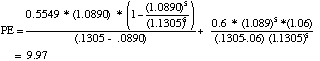B. P/E Ratio Based Upon Stable Growth (6%; 60% dividend payout)

= 0.6 * 1.06/(.1305 - .06) = 9.02

Difference Due to High Growth = 9.97 - 9.02 = 0.95

Question 4

A.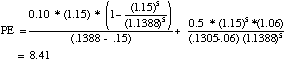B. Growth Rate from 1983 to 1993 = (0.78/0.08)(1/10) -1 = 25.57%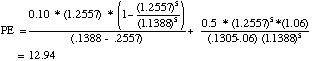C.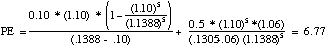Question 5

 A. South Korea Thailand Dividend Payout = 60% = 60% Expected Growth = (1.065)(1.058) - 1 = 12.68% =(1.075)(1.049) - 1 = 12.77% Cost of Equity = 12.90% + 7.5% = 20.40% = 15.50% = 8.00% + 7.50% P/E Ratio =0.6 * 1.1268/(.204-1268) = 8.75 =0.6 * 1.1277/(.1550-1277) = 24.76

*Expected Growth (in Nominal terms)

= (1+Expected Real Growth) (1+Exp. Inflation)

B. The regression yields the following:

P/E = 28.765 + 16.732 Interest Rate - 102.8 Inflation + 24.57 GDP Growth

R2=0.4175

C.

 Emerging Market P/E Ratio Predicted P/E P/E -Predicted China 18.0 16.99768 1.00 Hong Kong 18.1 24.46113 -6.36 India 26.6 22.41671 4.18 Indonesia 24.1 23.22113 0.88 Malaysia 34.6 28.26511 6.33 Philippines 21.5 22.43933 -0.94 Singapore 26.2 29.01850 -2.82 South Korea 22.0 26.56018 -4.56 Taiwan 34.0 28.30933 5.69 Thailand 23.5 26.91087 -3.41

Negative numbers suggest the market is undervalued. Positive numbers suggest overvaluation.

Question 6

A. Dividend Payout Ratio = 0.0274/(1/21.2) = 0.581

Cost of Equity = 6% + 5.5% = 11.5%

Solving for the Implied Growth Rate

g = (21.2 * .115 - 0.581)/(21.2 + .581) = 8.53%

1+g = (1+ Expected Inflation Rate) (1+ Real Growth Rate)

Solving for Expected Inflation

1.0853 = (1+Expected Inflation rate) (1.025)

Expected Inflation Rate = 1.0853/1.025 - 1 = 5.88%

B. The P/E ratio would go down. For instance, in the formulation above,

Dividend Payout Ratio = 0.581

Cost of Equity = 12.5%

Expected Growth Rate =8.53%

The new P/E ratio would be

P/E = 0.581 (1.0853)/(.125 - .0853) = 15.88

C. Not necessarily. If the increase in expected real growth is greater than the increase in interest rates, P/E ratios may go up as interest rates go up.

Question 7

A. Average P/E Ratio for the Industry = 13.2

Median P/E Ratio for the Industry = 12.25

If the firms in this group are homogeneous, the average P/E ratio provides an estimate of how much the market values earnings in this sector, given the expected growth potential and the risk in the sector.

The average P/E ratio can be skewed by extreme values (usually high, since P/E cannot be less than zero). The median corrects for this by looking at the median firm in the sector.

B. This statement is likely to be true only if

(1) Thiokol has the same growth prospects and risk profile of the typical firm in the industry. It also generates cash flows for disbursement as dividends which are similar to the typical firm in the industry.

(2) Thiokol has higher growth potential and/or lower risk than the typical firm in the industry.

C. The regression of P/E ratios on fundamentals yields the following:

P/E = -2.33 + 35.74 Growth Rate + 11.97 Beta + 2.90 Payout Ratio

R2= 0.4068

The following table provides predicted P/E ratios for the firms in the group:

 Actual P/E Predicted P/E Difference Boeing 17.30 12.90 4.40 General Dynamics 15.50 17.90 -2.40 GM- Hughes 16.50 13.68 2.82 Grumman 11.40 12.07 -0.67 Lockheed Corp. 10.20 12.31 -2.11 Logicon 12.40 13.17 -0.77 Loral Corporation 13.30 13.21 0.09 Martin Marietta 11.00 11.34 -0.34 McDonnell Doug. 22.60 17.15 5.45 Northrop 9.50 14.82 -5.32 Raytheon 12.10 10.85 1.25 Rockwell 13.90 14.85 -0.95 Thiokol 8.70 11.44 -2.74 United Industrial 10.40 9.11 1.29

Again, negative numbers indicate that the stock is undervalued.

The problem with a regression like this one is that it has relatively few observations and is likely to be thrown off by a few extreme observations.

Question 8

A. Expected Growth Rate = 25%

Unlevered Beta = 1.15/(1 + 0.6 * 0.25) = 1.00

FCFE = Net Income + Depreciation - Capital Spending = 10 + 5 - 12 = 3

Estimated Dividend Payout Ratio = 3/10 = 30%

P/E = 18.69 + 0.0695 * 25 - 0.5082 (1.00) - 0.4262 * 0.30 = 19.79

B.

1. The cross-sectional relationship between P/E ratio and the fundamentals may change over time.

2. The market might be overvaluing all stocks.

3. Some of the fundamentals, such as growth rate or beta, might be estimated with error.

Question 9

F. A firm with a lower P/E ratio than its peer group, a higher expected growth rate and lower risk.

Question 10

A. Expected Growth Rate = 10%

Cost of Equity = 7% + 0.8 * 5.5% = 11.4%

FCFE = Net Income + (Cap Ex - Depreciation) (1 - debt ratio)

+ Working Capital Change (1- Debt ratio)

= 120 - (80 - 77) * .9 - 15 * .9 = \$103.8 million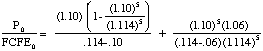= 23.24

B. EBIT = Net Income/(1-tax rate)+Interest Expenses

= 120/0.6 + 19 = \$219 million

FCFF = 219 * (1 - 0.4) - (80 - 77) - 15 = 113.4 million

Value of Firm = Value of Equity + Value of Debt

= 23.24 * 103.8 + 251 = \$2663 million

Value of Firm/FCFF = 2663/113.4 = 23.48

Value of Firm/EBIT = 2663/219 = 12.16 (lower because it is a pre-tax number)

Value of firm/EBITDA = 2663/(219+77) = 9.00 (lower because depreciation is added in while capital expenditures are ignored)

SOLUTIONS

PRICE/BOOK VALUE MULTIPLES

Question 1

A. False. If the ROE< Required rate of return, this can be justified.

B. False, since the drop can be temporary. If the drop is permanent, this will be generally true, since there will be a two-layered impact. The growth will go down, pushing down Price/Book value ratios. The ROE will also go down pushing P/BV ratios down even further.

C. True.

D. True. If other things (like risk) are not equal, this can be false.

E. False. The growth rate will be lower for these firms. The net effect may be a lower price/book value ratio.

Question 2

A. Dividend Payout Ratio = \$2/\$4 = 50%

Return on Equity = \$4/\$40 = 10%

Cost of Equity = 7% +0.85 * 5.5% = 11.68%

Expected Growth Rate = 6%

Price/Book Value Ratio = (.1) (.5)(1.06)/(.1168 - .06) = 0.93

A simpler solution might be the following:

Price/Book Value Ratio = (.10 - .06)/(.1168 - .06) = 0.70

(This solution takes into account the relationship between ROE and g, i.e., g=b(ROE))

B. If the P/BV ratio is 1.5, using the first approach,

1.5 = ROE (.5) (1.06)/(.1168 - .06),

Solving for ROE = 16.08%

Using the second approach,

1.5 = (ROE - .06)/(.1168 - .06)

Solving for ROE = 14.52%

Question 3

A. Average Price/Book Value Ratio = 2.28

Average ROE = 12.44%

Average Beta = 1.10

B. Cost of Equity (based upon average beta) = 7% + 1.1 * 5.5%

= 13.05%

If P/BV = (ROE - g)/(r - g),

and ROE < r, (as in this case)

then P/BV <1.

Therefore, one may conclude that stocks in the industry are, on average, overvalued relative to book value (assuming that the industry overall is in stable growth, although individual firms might still have extraordinary growth).

Question 4

A. Expected growth rate over next 5 years = ROE * (1- payout ratio) = 0.21* (1-.10) = 19.9%

Expected ROE after year 5 = g/ (1- ROE) = 0.06/(1-.4) = 0.15 or 15%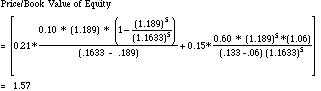B. Changed growth rate, keeping everything else constant.

 Growth rate P/BV 0.5 1.08 0.15 1.34 0.2 1.64 0.25 2 0.3 2.43 0.4 3.49 0.5 4.9

C. Between 12 and 13 years (this can be solved through trial and error).

Question 5

A.

 Next 10 yrs After yr 10 Payout Ratio 37.00% 60.00% Expected Growth 19.85% 6.00% Cost of Equity 12.88% 11.50% ROE 31.50% 15.00%

Expected Growth Rate = (1 - Payout Ratio)*ROE = (1 - .37) (.3150)

= .1985

Payout Ratio After Year 10 = 1 - Growth Rate / ROE

= 1 - 6%/15% = .60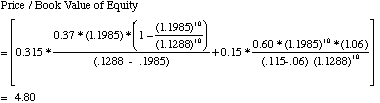B.

 Next 10 years After year 10 Payout Ratio 37.00% 60.00% Expected Growth 12.60% 6.00% Cost of Equity 12.88% 11.50% ROE 20.00% 15.00%

Expected Growth Rate = (1 - Payout Ratio)*ROE = (1 - .37) (.20)

= .126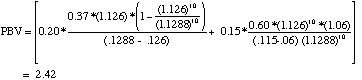Question 6

A.

 Year ROE g = b (ROE) Period of Growth P/BV 1988 27.00% 27.00% 10 2.55 1989 26.30% 26.30% 9 2.17 1990 23.90% 23.90% 8 1.68 1991 21.50% 21.50% 7 1.34 1992 18.30% 18.30% 6 1.07 1993 6.30% 6.30% 5 0.60

The price/book value ratio is estimated using a growth rate of 6% and a payout ratio of 50% after the period of high growth. The cost of equity used in the discounting is 13.6%.

B. No. The P/BV ratios are based upon expected returns on equity. These expectations may decline much more gradually than the actual ROE.

Question 7

A.

 Company Price BV/ P/BV Beta Exp. Payout ROE Share Growth Air & Water \$9.60 \$8.48 1.13 1.65 10.50% 0.00% 4.72% Allwaste \$5.40 \$3.10 1.74 1.10 18.50% 0.00% 8.06% Browning Ferris \$29.00 \$11.50 2.52 1.25 11.00% 46.90% 12.61% Chemical Waste \$9.40 \$3.75 2.51 1.15 2.50% 33.33% 12.00% Groundwater \$15.00 \$14.45 1.04 1.00 3.00% 0.00% 4.50% Intern'l Tech. \$3.30 \$3.35 0.99 1.10 11.00% 0.00% 4.78% Ionics Inc. \$48.00 \$31.00 1.55 1.00 14.50% 0.00% 7.10% Laidlaw Inc. \$6.30 \$5.85 1.08 1.15 8.50% 30.00% 6.84% OHM Corp. \$16.00 \$5.65 2.83 1.15 9.50% 0.00% 10.62% Rollins \$5.10 \$3.65 1.40 1.30 1.00% 0.00% 1.37% Safety-Kleen \$14.00 \$9.25 1.51 1.15 6.50% 45.00% 8.65% Average 1.66 1.18 8.77% 14.11% 7.39%

Dividend Payout = DPS/EPS

ROE = EPS/ BV of Equity

The average price/book value ratio of these firms is 1.66, based on the following:

(1) These firms have, on average, a lower growth rate than the firm being valued.

(2) The firm being valued has more free cash flows available for paying dividends than the average firm in the sector.

(3) The firm is unlevered. It should therefore have a lower beta.

B. What subjective adjustments would you make to the price/book value ratio for this firm and why?

On all three counts, a higher price/book value ratio should be used for this company.

C. P/BV = - 0.105 + 0.51 Beta - 4.24 Growth - 1.76 Payout + 24.15 ROE

R2= 0.84

The coefficients in this regression measure the change in P/BV ratio that would occur for a unit change in these variables. (Because of the multicollinearity, be cautious of reading too much into these coefficients.)

For the firm being valued,

Beta = 1.18/(1 + (1 - 0.4)(.20)) = 1.05 (The firm has no debt. Hence, the unlevered beta.)

Expected Growth Rate = 25%

Payout Ratio = (\$3.50 - \$2.50)/\$3.50 = 0.2857

ROE = \$3.50/20 = 17.50%

P/BV = -0.105 + 0.51 (1.05) - 4.24 (.25) -1.76 (0.2857) + 24.15 (.175)

= 3.09

Question 8

A. The R squared of the regression measures the goodness of fit of the regression. A high R squared would provide the user with more comfort with the predictions from using the regression.

B. P/BV = 0.88 + 0.82 (0.2857) + 7.79 (.25) - 0.41 (1.05) + 13.81 (.175) = 5.05

This regression uses the information in the entire cross-section, and hence might capture more of the differences across firms in other industries.

SOLUTIONS

PRICE/SALES MULTIPLES

Question 1

A. True. Revenues cannot be negative.

B. False. It depends upon the profit margins of a firm's particular business.

C. False. The expected growth may go up to offset the lost profit margins.

D. True.

E. False. It depends upon the prices you pay for the high profit margins.

Question 2

A. Dividend Payout Ratio = \$1.12/\$2.45 = 0.4571

Expected Growth Rate = 6%

Cost of Equity = 7% + 0.9 (5.5%) = 11.95%

Profit Margin = 2.45/122 = 2%

P/S Ratio = .02 * 0.4571 * (1.06)/(.1195 - .06) = 0.16288

Price Based on this Multiple = 0.16288 * 122 = \$19.87

B. P/S Ratio Needed for a Price of \$34 = \$34/122 = 0.2787

Profit Margin Needed for this P/S Ratio

= 0.2787 * (.1195 - .06)/(0.4571 * 1.06)

= 0.0342 or 3.42%

Question 3

A. These are the two companies with high expected growth rates. These high growth rates may explain the high P/S ratios. In addition, the Bombay company has the highest profit margin of the group.

B.

 Correlation between P/S ratio and profit margin = 0.8840 Correlation between P/S ratio and expected growth = 0.7694 Correlation between P/S ratio and beta = 0.2754 Correlation between P/S ratio and payout = -0.4390

C. One measure that might work is the ratio of Price/Sales (P/S) ratio to profit margin. On this basis, Bradlee's which has a P/S ratio of 0.09 and a profit margin of 1.04%, Caldor and Sears are most likely to be undervalued, whereas the Bombay company with P/S-Margin ratio of 0.56 is most likely to be overvalued.

 Company Price Sales P/S Ratio Profit Margin Exp. Growth Beta P/S- Bombay Co. \$38 \$9.70 3.92 7.01% 29.00% 1.45 0.559 Bradlees 15 168.6 0.09 1.04% 12.00% 1.15 0.086 Caldor 32 147.45 0.22 1.83% 12.50% 1.55 0.119 Consol. Store 21 23 0.91 4.13% 26.50% 1.35 0.221 Dayton Hudson 73 272.9 0.27 1.70% 12.50% 1.3 0.157 Federated 22 58.9 0.37 2.38% 10.00% 1.45 0.157 Kmart 23 101.45 0.23 1.72% 11.50% 1.3 0.131 Nordstrom 36 43.85 0.82 3.65% 11.50% 1.45 0.225 Penney 54 81.05 0.67 4.32% 10.50% 1.1 0.154 Sears 57 150 0.38 3.03% 11.00% 1.35 0.125 Tiffany's 32 35.65 0.9 4.21% 10.50% 1.5 0.213 Wal-Mart 30 29.35 1.02 3.58% 18.50% 1.3 0.286 Woolworth 23 74.15 0.31 1.82% 13.00% 1.25 0.17

Alternatively, a regression of P/S ratios against the fundamental variables could have been run and estimated P/S ratios can be obtained.

Question 4

A.

Profit Margin = 221/8298 = 2.66%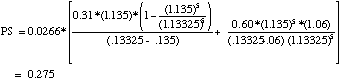B. P/S ratio for Stable Growth Firm with Same Margin

= 0.0266 * 0.6 * 1.06/(.13325 - .06) = 0.231

P/S ratio attributable to High Growth = 0.275 - 0.231 = 0.044

Question 5

A.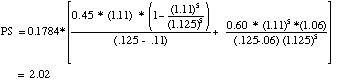B. New Margin = 100/700 = 14.29%

Old Growth Rate

= Old Profit Margin * Sales/Book Value * (1 - Payout ratio)

= .1784 * Sales/Book Value * (1 - 0.45) = 11%

Sales/Book Value = 1.12

New Growth Rate (for high growth period)

= .1429 * 1.12 * (1 - 0.45) = 8.81%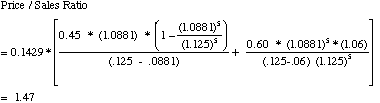Question 6

A. McDonald's

 Next 10 years After year 10 Payout Ratio 20.00% 65.00% Sales/Book Value 1.20 1.20 Expected Growth Rate 14.03% 6.00% Cost of Equity 12.775% 12.775% Profit Margin 14.61% 14.61%

Expected Growth Rate = (1 - Payout Ratio) * Profit Margin * Sales/BV

= (1 - 0.2) * .1461 * 1.2 = 14.03%

P/S Ratio = 1.97

Sales Per Share = 1.2 * 19 = \$22.80

Price Per Share = 1.97 * (\$22.80) = \$44.93

Wendy's

 Next 10 Years After Year 10 Payout Ratio 32.00% 65.00% Sales/Book Value 2.00 2.00 Expected Growth 8.30% 6.00% Cost of Equity 12.775% 12.775% Profit Margin 6.10% 6.10% P/S Ratio = 0.5711755 Price Per Share = \$8.00

B. If McDonald's sales/book value ratio remains unchanged and the profit margin drops to 6.10%:

 Next 10 Years After Year 10 Payout Ratio 20.00% 65.00% Sales/Book Value 1.20 1.20 Expected Growth Rate 5.86% 6.00% Cost of Equity 12.775% 12.775% Profit Margin 6.10% 6.10% P/S Ratio = 0.41691165 Price Per Share = \$9.51

C. Value of McDonald's with its Current Profit Margin = \$44.93

Value of McDonald's with Wendy's Profit Margin = \$ 9.51

Difference in Value Per Share = \$35.42

Value of Brand Name = \$35.42 * Number of Shares Outstanding =

= 35.42 * (7.425/(19 * 1.2)) = \$11.54 Billion

Question 7

A.

 Next 10 Years After Year 10 Payout Ratio 33.00% 60.00% Sales/Book Value 2.50 2.50 Expected Growth Rate 16.75% 6.00% Cost of Equity 14.15% 12.50% Profit Margin 10.00% 10.00% P/S Ratio = 1.59991143 Price per share = \$39.00

B.

 Next 10 Years After Year 10 Payout Ratio 33.00% 60.00% Sales/Book Value 3.00 3.00 Expected Growth Rate 16.08% 6.00% Cost of Equity 14.15% 12.50% Profit Margin 8.00% 8.00% P/S Ratio = 1.21549194 Price Per Share = \$35.55

C. The status quo strategy is best, since it leads to a higher price per share.

D. Sales would have to drop 20%. (Sales/book value ratio would have to be 2.40 for the two strategies to be equivalent.)

Question 8

A. No. One would explain its high price to sales ratio by pointing to the combination of a high profit margin and a moderate growth rate.

B. P/S = -0.79 + 11.50 Profit Margin + 0.60 Payout + 1.50 Growth + 0.51 Beta

Walgreen's predicted P/S ratio would be -

P/S = -0.79 + 11.50 (0.027) + 0.60 (.31) + 1.50 (.135) + 0.51 (1.15) = 0.4955

The small number of observations in this regression is of concerns, as are the signs on the coefficients for the variables. To the extent that the regression assumes that the relationship between P/S ratios and the fundamentals will not change, shifts in these relationships would also be cause for concern.

C. P/S = -0.79 + 11.50 (0.06) + 0.60 (0) + 1.50 (.20) + 0.51 (0.93) = 0.67

(The average beta for the industry was used, since this firm will have the same debt ratio as the average firm.)

Question 9

A. The coefficients on this regression measure both the direction and the magnitude of the relationship between P/S ratios and independent variables. My concerns would be the same as for the peer group regression.

B.

 Company P/S Ratio Profit Margin Payout Exp. Growth Beta Predicted P/S Arbor Drugs 0.42 3.40% 18% 14.00% 1.05 0.39904 Big B Inc. 0.30 1.90% 14% 23.50% 0.70 0.48704 Drug Emporium 0.10 0.60% 0% 27.50% 0.90 0.28121 Fay's Inc. 0.15 1.30% 37% 11.50% 0.90 0.34188 Genovese 0.18 1.70% 26% 10.50% 0.80 0.37292 Longs Drug 0.30 2.00% 46% 6.00% 0.90 0.38680 Perry Drugs 0.12 1.30% 0% 12.50% 1.10 0.14108 Rite Aid 0.33 3.20% 37% 10.50% 0.90 0.48487 Walgreen 0.60 2.70% 31% 13.50% 1.15 0.33992

These predictions use the information in the entire cross-section, and should be more reliable.

C. P/S = 0.42 + 0.33 * 0 + 0.73 * 0.20 - 0.43 * 0.93 + 7.91 * 0.06

= 0.64

The values in this regression are the values of the private firm being valued.

Market Value of Equity = Revenues * Price/Sales Ratio

= 250 * 0.64 = \$160 million